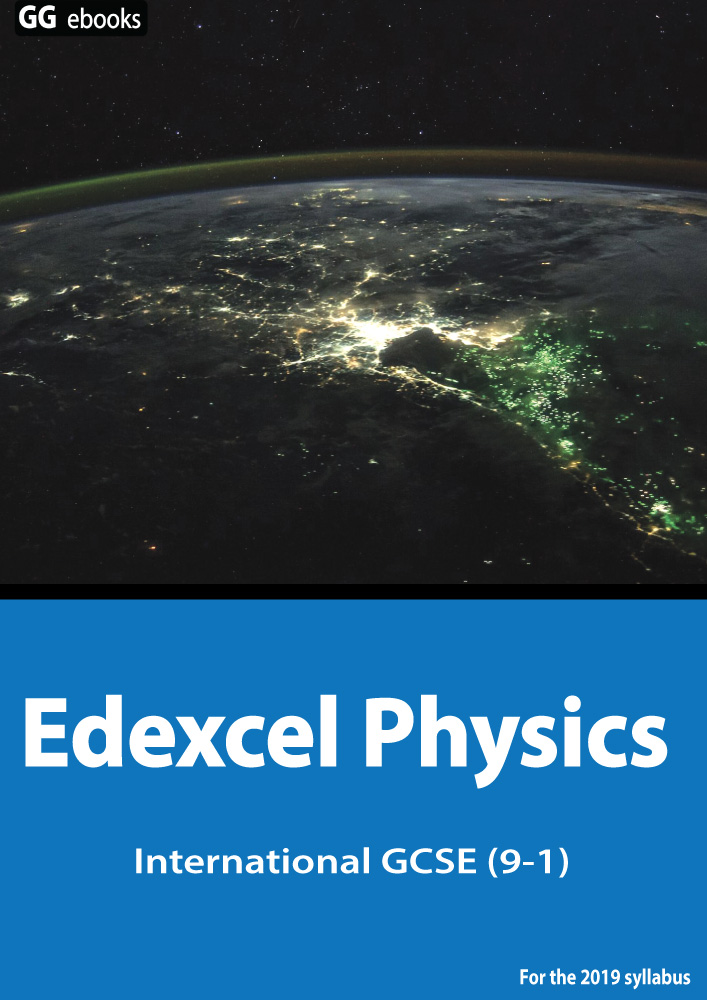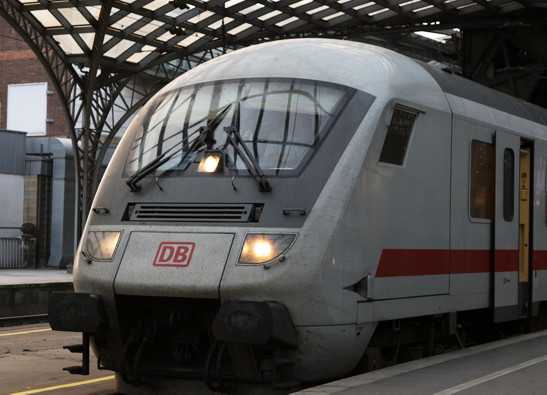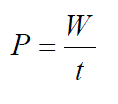Edexcel IGCSE - Energy

Page 1

 NEED HELP? Edexcel iGCSE digital textbooks From the makers of GradeGorilla. GGebooks.com (Opens in a new tab)1. Work done is measured in

• A) joules
• B) watts
• C) coulombs
• D) newtons

2. Which of these is NOT a store of energy?

• A) chemical
• B) gravitational
• C) friction
• D) kinetic

3-8. Choose the correct energy store in the following systems:

 A bow and arrow transfers ? thermal kinetic gravitational nuclear chemical elastic energy into useful ? thermal kinetic gravitational nuclear chemical elastic energy. A petrol engine in a car transfers ? thermal kinetic gravitational nuclear chemical elastic energy into useful ? thermal kinetic gravitational nuclear chemical elastic energy. A falling ball transfers ? thermal kinetic gravitational nuclear chemical elastic energy into ? thermal kinetic gravitational nuclear chemical elastic energy.

A motor on a train converts 60 000J of electrical energy into 45 000J of kinetic energy as the train accelerates.9. How efficient is the motor?
10. If it converts this energy in 15 seconds, what is the useful power output?11. What is the formula for work done?

• A) Work done = force x distance
• B) Work done = force ÷ distance
• C) Work done = mass x acceleration
• D) Work done = mass ÷ acceleration

12. A tractor pulls a plough 2km across a field using a force of 8kN. What is the work done?